Students can download Maths Chapter 2 Integers Ex 2.2 Questions and Answers, Notes, Samacheer Kalvi 6th Maths Guide Pdf helps you to revise the complete Tamilnadu State Board New Syllabus, helps students complete homework assignments and to score high marks in board exams.

## Tamilnadu Samacheer Kalvi 6th Maths Solutions Term 3 Chapter 2 Integers Ex 2.2

Miscellaneous Practice Problems

Question 1.
Write two different real-life situations that represent the integer -3.
Solution:
(i) A sapling planted at a depth of 3m
(ii) Sheela lost ₹ 3 on selling an apple.Question 2.
Mark the following numbers on a number line.
(i) All integers which are greater than -7 but less than 7.
(ii) The opposite of 3.
(iii) 5 units to the left of -1.
Solution: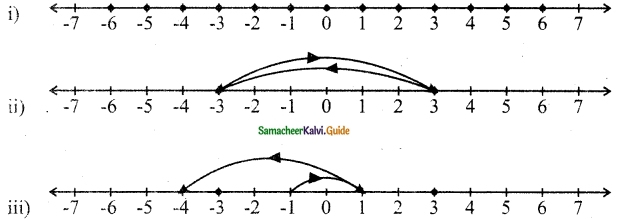Question 3.
Construct a number line that shows the depth of 10 feet from the ground level and its opposite.
Solution: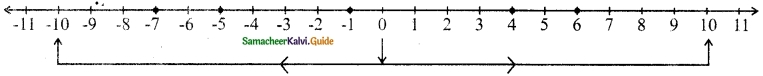Question 4.
identify the integers and mark on the number line that are at a distance of 8 units from – 6.
Solution: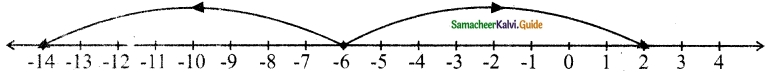Question 5.
Answer the following questions from the number line given below.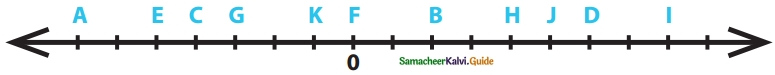(i) Which integer is greater: G or K? Why?
(ii) Find the integer that represents C
(iii) How many integers are there between G and H?
(iv) Find the pairs of letters which are opposite of a number,
(v) Say True or False: 6 units to the left of D is -6.
Solution:
(i) K is greater. K represents -1 and G represents -3. Because it is to the right of G in the negative side of the number line.
(ii) C represents -4
(iii) G represents -3 and H represents 4.
∴ -2, -1, 0, 1, 2, 3 are the 6 numbers between G and H.
(iv) (C, H) and (E, J) are opposite pairs.
(v) False. 6 units to the left of D is 0. Because D represents +6 on the number line

Question 6.
If G is 3 and C is -1, what numbers are A and K on the number line?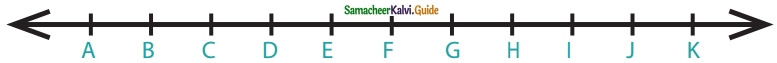Solution:
A (-3), K (7)Question 7.
Find the integers that are 4 units to the left of 0 and 2 units to the right of -3?
Solution:
-4, -1

Challenge Problems

Question 8.
Is there the smallest and the largest number in the set of integers? Give reason.
Solution:
No, we cannot find the smallest (-) and largest (+) number in the set of integers, as the numbers on the number line extend on both sides without an end.

Question 9.
Look at the Celsius Thermometer and answer the following questions.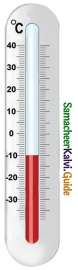(i) What is the temperature that is shown in the Thermometer?
(ii) Where will you mark the temperature 5°C below 0° C in the Thermometer?
(iii) What will be the temperature, if 10° C is reduced from the temperature shown in the Thermometer?
(iv) Mark the opposite of 15° C in the Thermometer.
Solution:
(i) – 10°C
(ii) – 5°C
(iii) -20°C
(iv) -15°CQuestion 10.
P, Q, R, and S are four different integers on a number line. From the following clues, find these integers and write them in ascending order.
(i) S is the least of the given integers.
(ii) R is the smallest positive integer.
(iii) The integers P and S are at the same distance from 0.
(iv) Q is 2 units to the left of integer R.
Solution:
S < Q < 0 < R < P

Question 11.
Assuming that the home to be the starting point, mark the following places in order on the number line as per instruction given below and write their corresponding integers.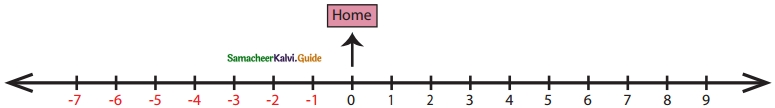Places: Home, School, library, Playground, Park, Departmental Store, Bus stand, Railway Station, Post Office, Electricity Board.
Instructions:

1. The bus stand is 3 units to the right of the Home.
2. The library is 2 units to the left of Home.
3. Departmental Store is 6 units to the left of Home.
4. The post office is 1 unit to the right of the Library.
5. Park is 1 unit right of Departmental Store.
6. Railway Station is 3 units left of Post Office.
7. Bus Stand is 8 units to the right of Railway Station.
8. School is next to the right of the Bus Stand.
9. Playground and Library are opposite to each other.
10. Electricity Board and Departmental Store are at equal distance from Home.

Solution:

1. 3
2. -2
3. -6
4. -1
5. -5
6. -4
7. 4
8. 4
9. 5
10. 2Question 12.
Complete the table using the following hints.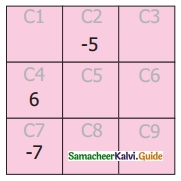(i) C1 : the first non-negative integer.
(ii) C3 : the opposite to the second negative integer.
(iii) C5 : the additive identity in whole numbers.
(iv) C6 : the successor of the integer in C2.
(v) C8 : the predecessor of the integer in C7.
(vi) C9 : the opposite to the integer in C5.
Solution:
(i) C1 : (0)
(ii) C3 : (2)
(iii) C5 : (0)
(iv) C6 : (-4)
(v) C8 : (-8)
(vi) C9 : (0)Question 13.
The following bar graph shows the profit (+) and loss (-) of a small scale company (in crores) between the year 2011 to 2017.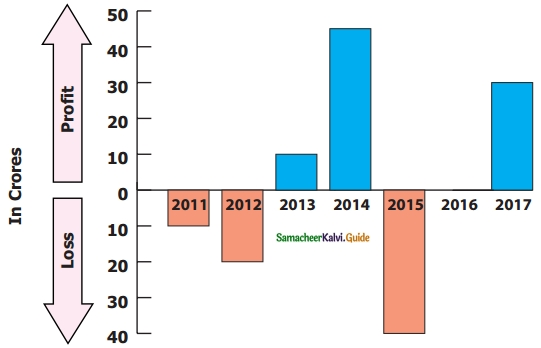(i) Write the integer that represents a profit or a loss for the company in 2014?
(ii) Denote by an integer on the profit or loss in 2016.
(iii) Denote by integers on the loss for the company in 2011 and 2012.
(iv) Say True or False: The loss is minimum in 2012.
(v) Fill in: The amount of loss in 2011 is _____ as profit in 2013.
Solution:
(i) Profit ₹ 45 crores. ∴ Ans : + 45
(ii) In 2016 neither profit nor loss happened. ∴ Ans : 0
(iii) In 2011 loss is 10 crores and in 2012 loss is 20 crores.
∴ -10 and-20.
(iv) False. In 2011 the company’s loss is minimum.
(v) The same. Because in 2013 the profit is 10 crores and in 2011 the loss is 10 crores.